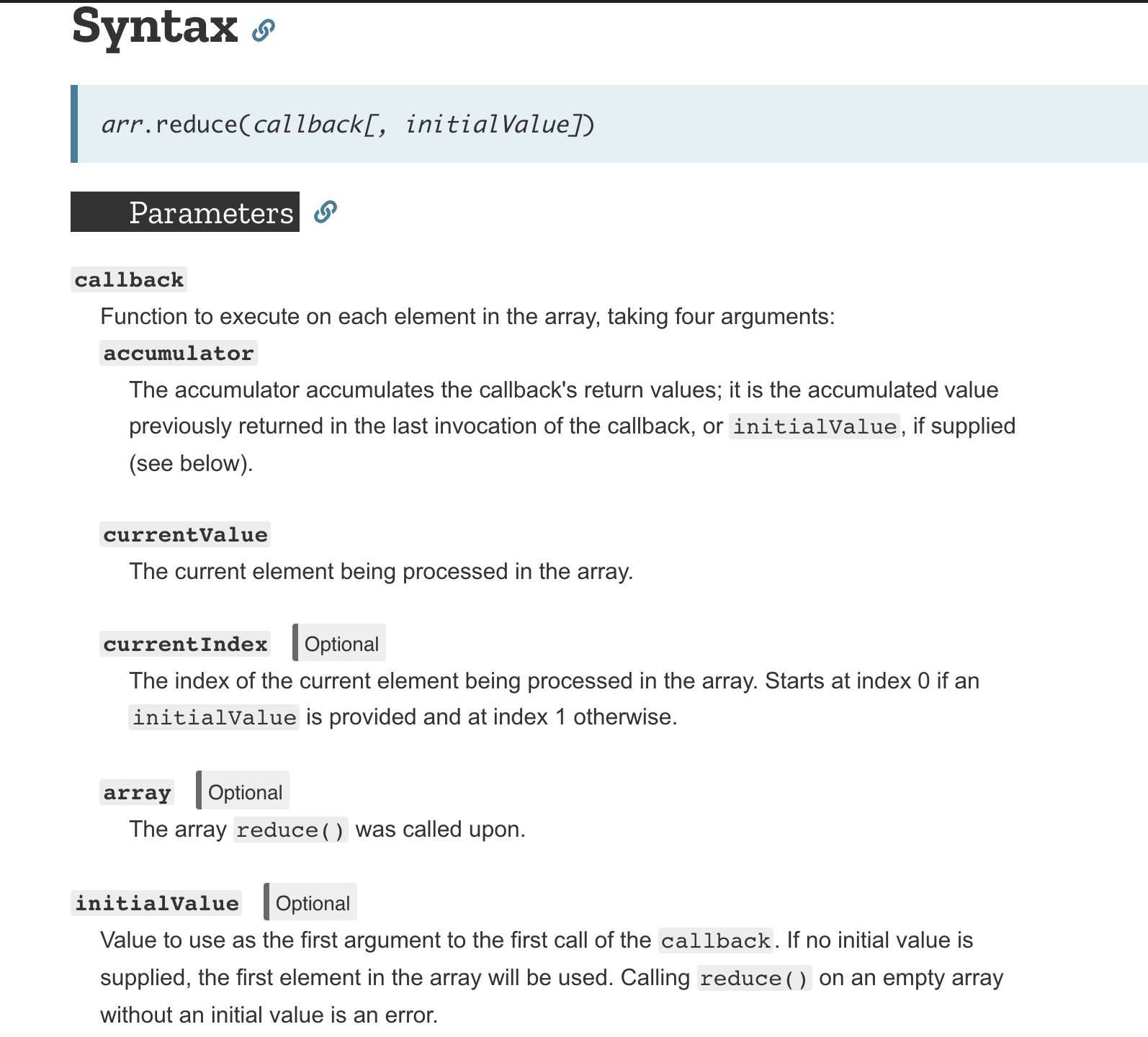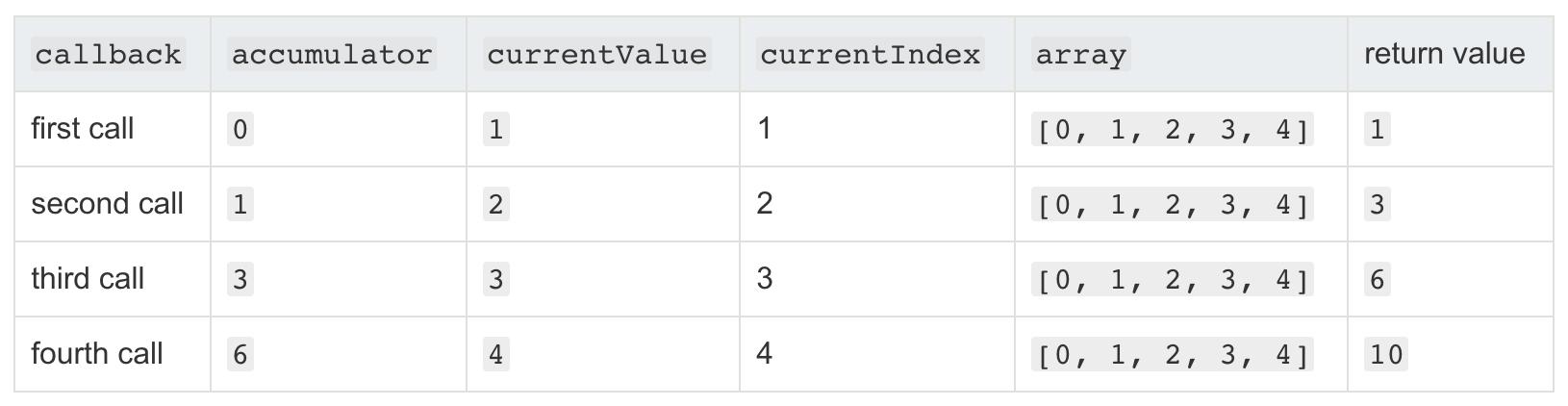Looking for a fancier way to add up all the numbers in an array? You might want to give `.reduce()` a try.

``````let arr = [0, 1, 2, 3, 4];

function getSum(total, num) {
}
console.log(arr.reduce(getSum)); // outputs 10
``````

Example from W3School and MDN

The .reduce() method (function that is inside an object in JavaScript), by definition, is to reduce an array into a single value.Fig. 1; from MDN

However, the execution is somewhat less than intuitive (to me, at least) by just looking at the raw code and the doc. Thus comes my version of interpretation on .reduce(). Here in part 1 I am only going through its callback, accumulator, and currentValue parameter, for the sake of simplicity. Now, let’s dissect the example code into two parts:

``````function getSum(total, num) {
}
``````

The first part is where we define the callback function[¹] (function that gets called after being defined) for later use in .reduce(). Inside of it we have the accumulator (total in example), the container that stores the sum of the previous values in each iteration, and currentValue (num), the number to be added in current cycle. The two works like this when the callback is called in .reduce():Fig. 2; from MDN

``````console.log(arr.reduce(getSum));
``````

We call “.reduce()” on an array (arr in example), with our callback `getSum()`. It should print out “10” the number without a problem.

A more thorough guide on initialValue and currentIndex parameter will be given in the next part.

[¹] You could give the callback function a name of your liking, but keeping it readable goes a long way